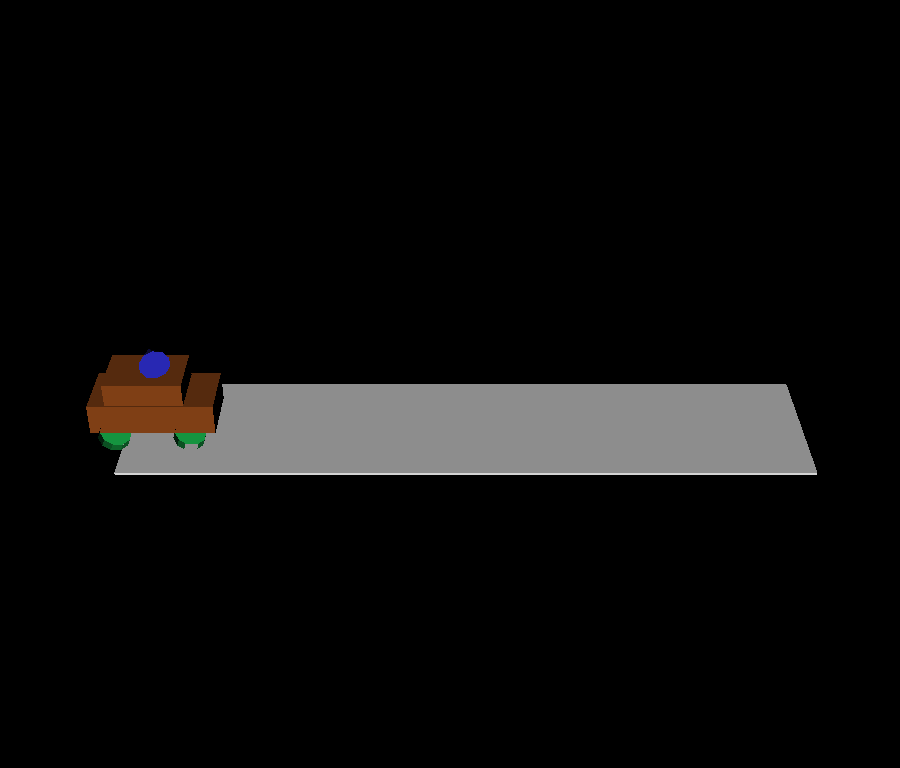# Keyframe hierarchical Animation#

Creating hierarchical keyframes animation in fury

```import numpy as np

from fury import actor, window
from fury.animation import Animation

scene = window.Scene()

showm = window.ShowManager(
scene, size=(900, 768), reset_camera=False, order_transparent=True
)
showm.initialize()
```

```road = actor.box(
np.array([[0, 0, 0]]), colors=np.array([[1, 1, 1]]), scales=np.array([[22, 0.1, 5]])
)
```

Constructing the car geometry

```body_actor = actor.box(
np.array([[0, 0.5, 0], [-0.2, 1, 0]]),
scales=((4, 1, 2), (2.5, 1.5, 1.8)),
colors=(0.6, 0.3, 0.1),
)
```

Adding the the car’s body to an Animation to be able to animate it later.

```car_anim = Animation(body_actor)
```

Creating the wheels of the car

```wheel_center = np.array([[0, 0, 0]])

wheel_direction = np.array([[0, 0, 1]])
wheel_positions = [
[1.2, 0, 1.1],
[-1.2, 0, 1.1],
[1.2, 0, -1.1],
[-1.2, 0, -1.1],
]

wheels = [
actor.cylinder(
wheel_center,
wheel_direction,
(0.1, 0.7, 0.3),
heights=0.3,
resolution=10,
capped=True,
)
for _ in range(4)
]
```

Animating each wheel and setting its position to the right position using a single keyframe that will not change.

```wheels_animations = [Animation(wheel) for wheel in wheels]

for wheel_anim in wheels_animations:
wheel_anim.set_position(0.0, wheel_positions.pop())
wheel_anim.set_rotation(0.0, [0, 0, 1, 1])
wheel_anim.set_rotation(1.0, [0, 0, 1, -1])
```

Creating a radar on top of the car

First we create the shaft holding and rotating the radar

```radar_shaft = actor.cylinder(
np.array([[0, 0, 0]]), np.array([[0, 1, 0]]), (0, 1, 0), heights=1
)
```

In order to animate the shaft actor we have to add it to an Animation

```radar_shaft_anim = Animation(radar_shaft)
```

Setting a single position keyframe will make sure the actor will be placed at that position

```radar_shaft_anim.set_position(0.0, [0, 2, 0])
```

Rotating the shaft around Y axis

```radar_shaft_anim.set_rotation(0.0, [0, -250, 0])
```

Now we create the radar itself

```radar = actor.cone(np.array([[0, 0, 0]]), directions=(0, 0, 0), colors=(0.2, 0.2, 0.9))
```

Then add it to an animation in order to rotate it

```radar_animation = Animation(radar)
```

Set position and rotation as done above with the shaft.

```radar_animation.set_position(0, [-0.4, 0.5, 0])
```

Now, we want the radar to rotate when the shaft rotates in hierarchical way. To do that we must add the radar animation as a child animation of the shaft animation as below:

```radar_shaft_anim.add_child_animation(radar_animation)
```

After that we want everything to animate related to the car. The wheels should always be attached to the car no matter where it moves. we do that by adding them as child animations of the car’s body animation

```car_anim.add_child_animation([wheels_animations, radar_shaft_anim])
```

Moving the car

```car_anim.set_position(0.0, [-10, 0.5, 0])
car_anim.set_position(6.0, [10, 0.5, 0])
```

Adding the car Animation to the show manager

```showm.add_animation(car_anim)
scene.camera().SetPosition(0, 20, 30)

interactive = False

if interactive:
showm.start()

window.record(
scene, out_path='viz_keyframe_hierarchical_animation.png', size=(900, 768)
)
```Total running time of the script: ( 0 minutes 0.101 seconds)

Gallery generated by Sphinx-Gallery